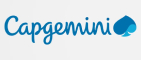New update is available. Click here to update.
Last Updated: 17 Dec, 2020

# Implement strStr()

Moderate+11 more companies

## Problem statement#### You are given two strings A and B. Find the index of the first occurrence of A in B. If A is not present in B, then return -1.

##### For Example:
``````A = “bc”, B = “abcddbc”.
String “A” is present at index 1, and 5(0-based index), but we will return 1 as it is the first occurrence of “A” in string “B”.
``````
``````Can you solve this in linear time and space complexity?
``````
##### Input format:
``````The first line contains an integer 'T' which denotes the number of test cases or queries to be run. Then, the T test cases follow.

The first and only line of each test case contains two strings A and B, separated by a single space.
``````
##### Output format:
``````For each test case, print the index of the first occurrence of A in B, if string A is not present in string B then print -1.
``````
##### Note:
``````You do not need to print anything. It has already been taken care of. Just implement the given function.
``````
##### Constraints:
``````1 <= T <= 100
1 <= |A|, |B| <= 5 * 10^4

Time limit: 1 second
``````## Approaches

### 01 Approach• If the length of B is less than the length of A, then we simply return -1.
• Now let’s denote the length of A as M and the length of B as N.
• Now, we run a loop from 0 to N - M and for each index i in the range of 0 to N - M, we run another loop from 0 to M. Let’s denote this inner loop counter as j.
• Then match the characters at (i + j)th index of B with (j)th index of A. If at any point characters mismatch, then we break the inner loop and increment i by 1. If all these characters match, and we are out of the inner loop, then we return i, as it is the index of the first occurrence of A in B.
• If we reach at the end of the outer loop, then we return -1, as A is not present in B.